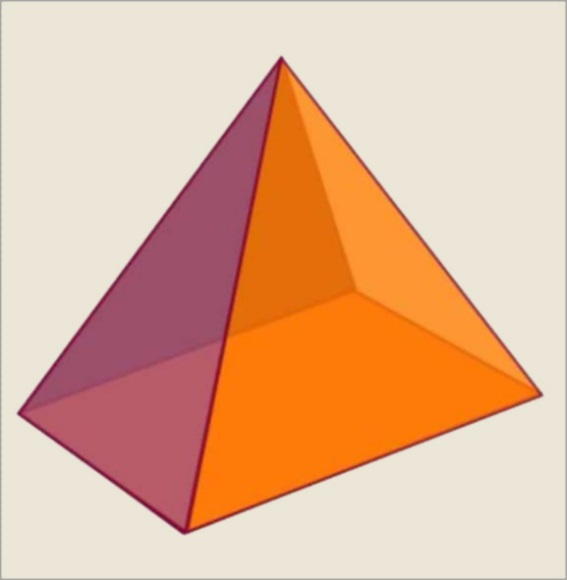Three Dimensional Shapes - 3D

Three Dimensional Shapes - Properties of 3D Shapes with Curves

Shape:

A shape can be defined as the form of an object or its outline or outer boundary. The different basic shapes are two-dimensional such as square, rectangle etc and three-dimensional shapes such as prism, cylinder, cone etc.

Three-dimensional shapes are that having three dimensions such as height, width, and length, like any object in the real world. It is also known as 3D.

Properties of 3D shapes:

• • Faces: A face is a curved or flat surface on a three-dimensional shape. For example: a cylinder has three and a sphere has just one, a cube has 6 faces.

• • Edges: An edge is where two faces meet/intersect. For example: a cylinder has two and a sphere has none, a cube has 12 edges.

• • Vertices: A vertex is a corner where edges join.

• Three-dimensional shapes, by nature they have an inside and outside, separated by a surface. All physical things that we touch are three dimensional.

Polyhedrons:

Polyhedral or polyhedrons are straight-sided solid shapes. They are based on polygons, two- dimensional plane shapes with straight lines.

Polyhedrons are defined as having straight edges, corners called vertices, and flat sides called faces.

The polyhedrons are often defined by the number of edges, faces and vertices, as well as their faces are all same size and same shape. Like polygons the polyhedrons can be regular and irregular based on regular and irregular polygons. Polyhedrons can be convex or concave.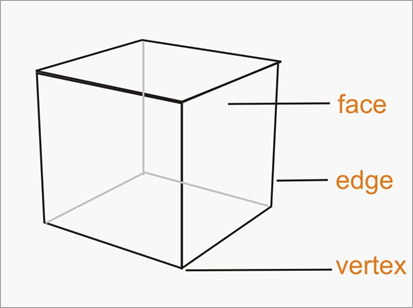Cube is the most familiar and basic polyhedron. It has 6 square faces, 12 edges and eight vertices.

Regular polyhedrons: (platonic solids)

The five regular solids are a special class of polyhedrons; all of the faces are identical with each face being a regular polygon.

The platonic solids are as below:
• • Tetrahedron: It has four equilateral triangle faces.

• Tetrahedron is a solid that include 4 plane triangular faces. It is also known as triangular pyramid.

Properties of tetrahedron:

• • The tetrahedron has four faces all of which are equilateral triangles.

• • There are 6 edges in the regular tetrahedron and all are equal in length.

• • A tetrahedron circumscribes/limit a sphere that is tangent to all the faces of the tetrahedron.

• • It can be inscribed in a sphere that passes through all the vertices.

• • The centre of the inscribed sphere, centre of the regular tetrahedron, and centre of circumscribing sphere itself are coincidence.

•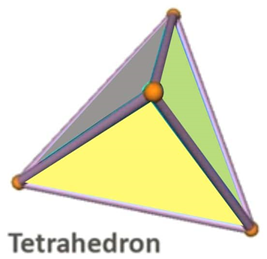• • Cube: It has six square faces.

• Cube is a symmetrical three-dimensional shape, either hollow or solid, contained by six equal squares. Three edges connect to each corner to form a vertex. The cube can be called a regular hexahedron also.

Properties of cube:

• • Face of the cube is also called as sides or facets. It has 6 faces which are all squares. Each face has four equal sides and all the four interior angles are right angles.

• • A cube has 12 edges. A line segment is formed where the two edges meet. The faces are square and congruent to each other. All the 12 edges are in the same length.

• • A cube has 8 vertices and the vertex is a point where three edges meet.

• • Face diagonals are line segments that link the opposite corners of a face. The face diagonals are two for each face and there will be total of 12 face diagonals in a cube.

• • Space diagonals are also line segments that link the opposite corners of the cube cutting through its interior. It has 4 space diagonals.

•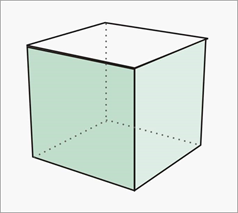The surface area of a cube is the area of one face that is length × width multiplied by 6 as all the faces are same.
If one face of a cube is 10 × 10 =100cm2, then multiply by 6 that is the number of faces in a cube then the surface area of the cube = 600cm2

• Octahedron: it is with eight equilateral triangle faces.

• It is a three-dimensional shape having eight plane faces, especially a regular solid figure with eight equal triangular faces.

Properties of octahedron:
• • The Base of the octahedron is a square.

•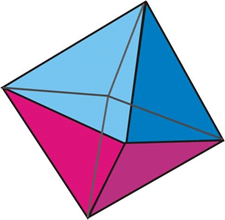Let’s have a look at the picture, the two congruent square pyramids have their bottom touching, then the base is the square between the two pyramids.

• • The Octahedron has about 8 faces, which are all in shape of the equilateral triangles. The eight faces are those solid gets its name “Octa”. Octa means eight. These faces form the surface area of the octahedron. The square that is the base of the solid is not part of the surface area. Therefore, the base is also not a face.

• • When the two faces touch the line segment is formed and it is called the edge. It has 12 edges.

• • When the two edges intersect they form the vertex. It has 6 vertices. Each of the vertexes is formed when four edges intersect.

• Dodecahedron: It is with twelve pentagon faces:

• Dodecahedron is a three-dimensional shape having twelve plane faces, a regular solid figure with twelve equal pentagonal faces.

Properties of the dodecahedron:

• • The dodecahedron has about 12 equal pentagonal faces, in which the Pentagon is a two- dimensional shape with s straight sides and 5 vertices. So a dodecahedron has 12 equal pentagonal faces.

•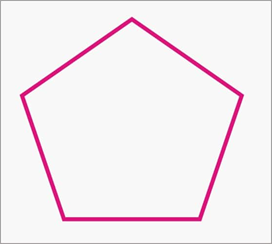• • The dodecahedron has 30 edges and the vertices are about 20 vertices.

•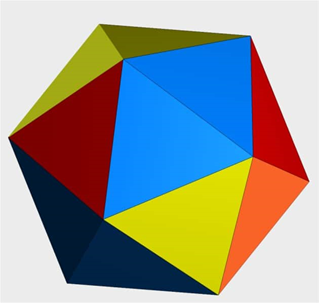• Icosahedron: with about twenty equilateral triangle faces.

• It is a solid figure with twenty plane faces, especially equilateral triangular ones.

Properties of Icosahedron:

• • It has 20 equilateral triangle faces i.e. 20 flat sides.

• • And have about 12 vertices and 30 edges.

•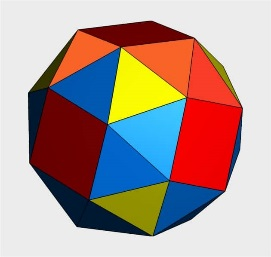Three-dimensional shapes with curves:

The solid shapes either which include the curved or round edge are not polyhedrons. They can only have straight lines.
Many objects around us will include at least some curves. The most common curved solids are cones, cylinder, sphere, and torus.

The common three-dimensional shape curves are the cylinder, cone, sphere, and torus.

Cylinder:

A cylinder is solid with a circular base. The cylinder will have the same cross-section from the one end to the other end. They have two identical ends of either an oval or a circle. Although the cylinders are similar to prisms, the prisms will have flat sides and parallelogram.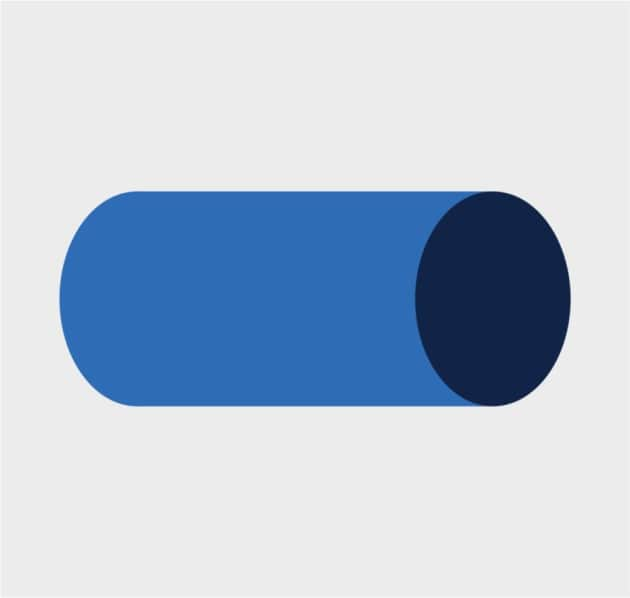Cone:

The cone has an oval or circular base and an apex or vertex. The side of the cone tapers smoothly to the vertex. The cone has a distinct single curved side and a circular base.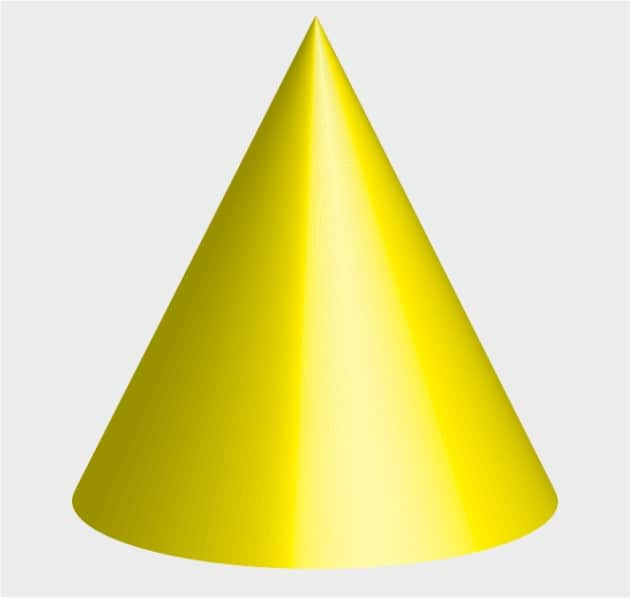Sphere:

The sphere is shaped like a ball or a globe. It is completely round. Every point on the surface of the sphere is an equal distance to the centre.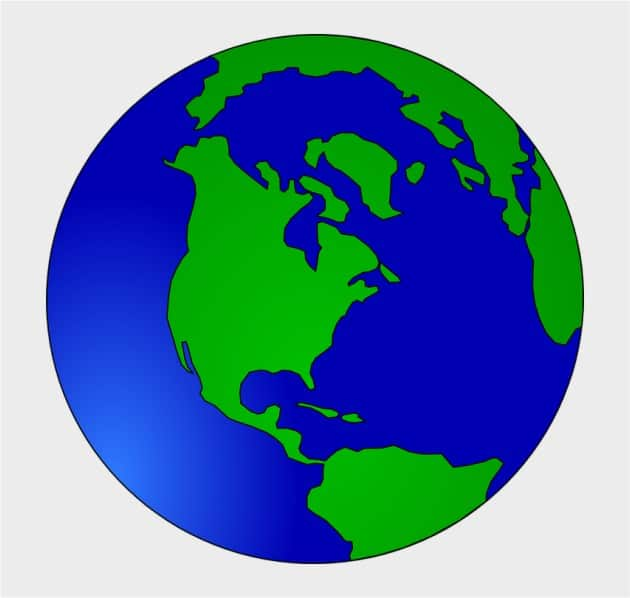Torus:

It is shaped like a ring or doughnut or tire. The torus is formed by revolving the smaller circle around the larger one. There is also a more complex form of torus.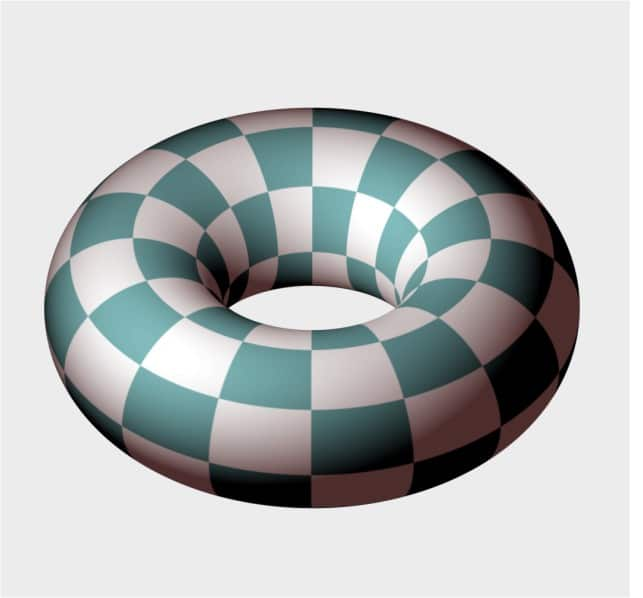What is a prism and pyramid?

Prism:

The prism is any polyhedron and has flat sides and matching ends. If a prism is cut anywhere along with its length, parallel to an end, its cross section will be the same and there will be two prisms. The sides of prisms are parallelograms, four-sided shapes with two pairs of sides with equal length.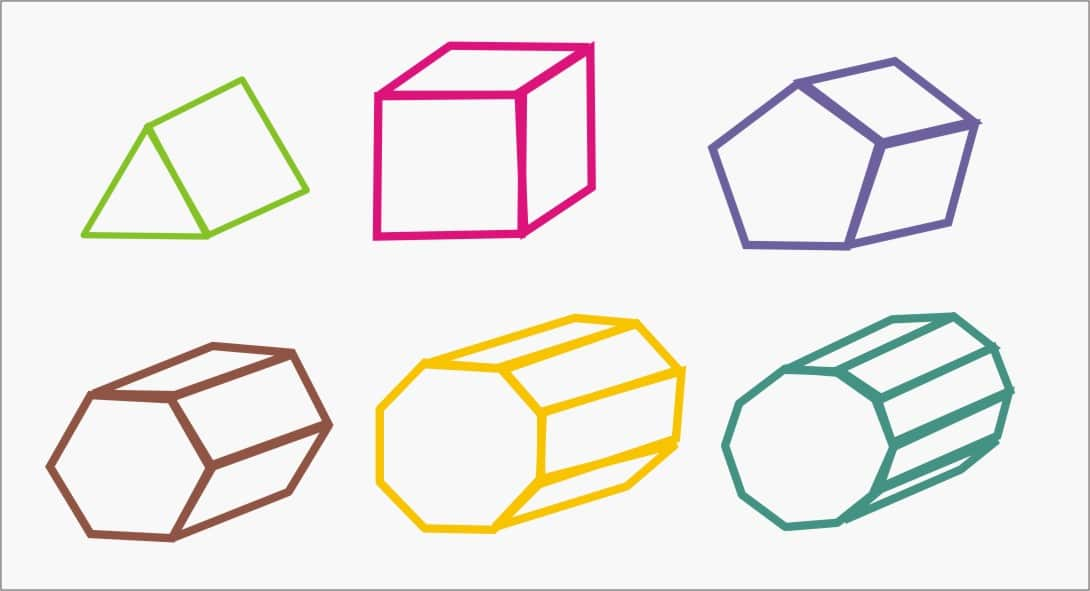These are different types of prisms.

The types of prisms are Regular and Irregular prisms.

Regular prism:

Regular prism is the one with regular polygon bases. The height of the prism is the distance between the bases.

Irregular prisms:

Irregular prisms have the irregular bases and the faces are not equally sized.
The volume of the prism = area of the base × height of the prism.

Altitude of a prism:

It is a line joining the two bases of the prism. The height is also called as the altitude or the shortest distance between the two bases of a prism. It can also be defined as the perpendicular segment that joins the 2 bases.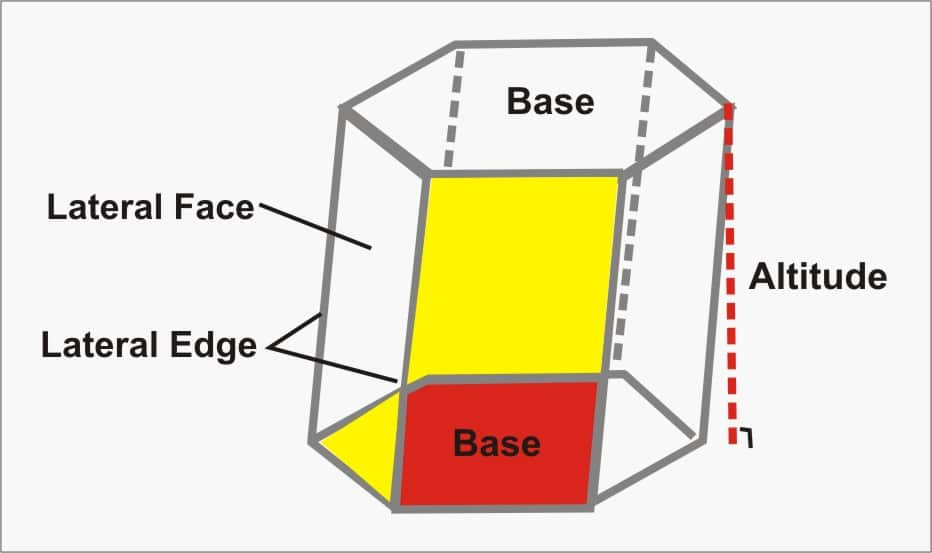Antiprisms are those like the regular prisms their ends would match. However antiprisms are made up of triangles and not parallelograms. Antiprisms can become more and more complex.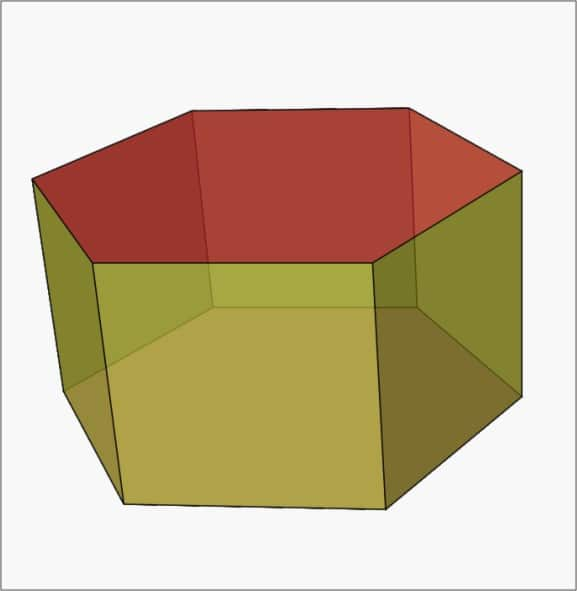Pyramid:

The Pyramid is a polyhedron with a polygon base that connects the top point i.e. the vertex with the straight sides. In many pyramids the lateral faces will also be congruent. Congruent shapes are those which are in same shape and size. The vertex/apex in the pyramid where the lateral faces meet.

Triangular pyramid:

A pyramid can have an apex in the direct centre of the base, is called a right pyramid. The triangular pyramid has a triangle for the base and three other sides which are also triangular. Therefore it has 4 faces, 4 vertices, and 6 edges.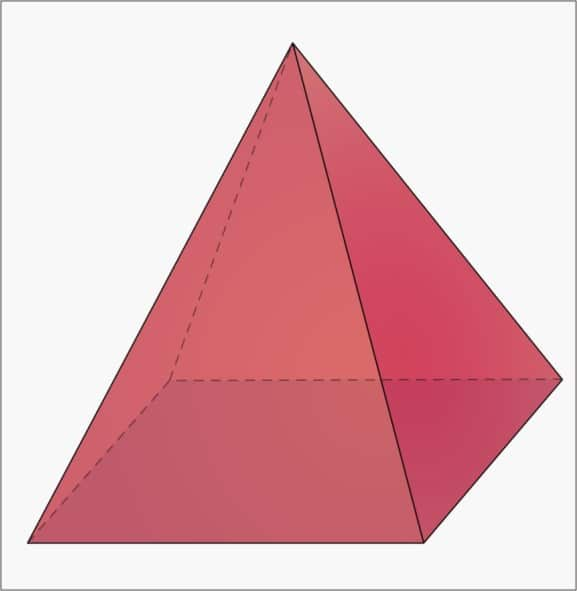Oblique pyramid:

The pyramid with the apex in the off centre is called the oblique pyramid. In simple terms, the oblique pyramid is one where the vertex or apex is not over the centre of the base.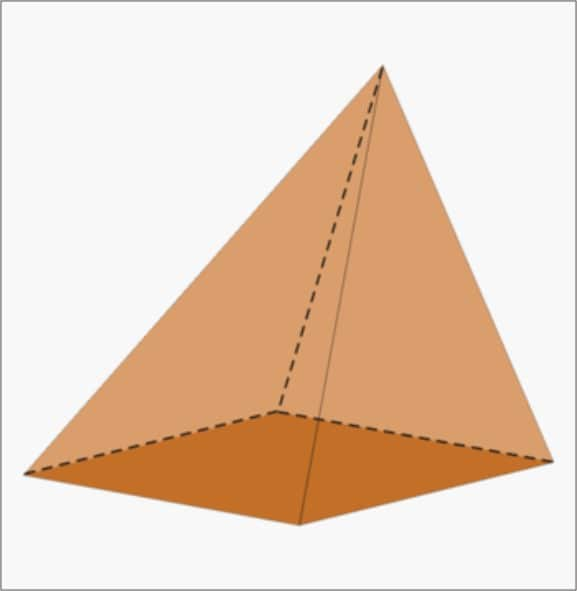Rectangular pyramid:

The rectangular pyramid has the base of a rectangle and the other four faces being triangles. The faces that are not rectangle but triangle are called lateral faces and meet the point called the vertex.